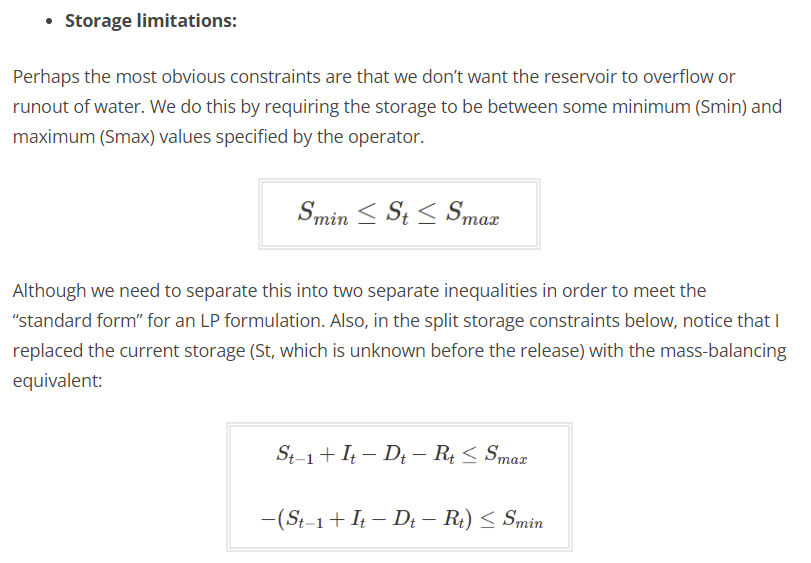## Thursday, October 5, 2023

### 1. Inventory balance constraints

I came accross this text :

Here $$S_t$$ is the reservoir storage level. This is just a fairly standard inventory balance constraint. We use this all the time in LP/MIP models. So actually we can use this equation as a constraint. This misunderstanding of LP modeling leads to more problematic modeling constructs.

### 2. Substituting out variables

Instead of bounds on $$S_t$$ they come up with:Simple bounds rewritten as more complex constraints.

This is exactly what we don't want to see: duplication of expressions. In large-scale modeling, substituting out variables is often a bad idea: it can destroy the sparsity of model. More importantly, it hurts the readability of the model.

### 3. Variables vs Parameters

In the table

all quantities are referred to as variables. In modeling, we make an important distinction between  variables (endogenous with values to be determined by the solver) and parameters (exogenous or data: constants during the solve). Just calling everything "variables" is wrong.

### 4. Single period vs multi-period models

Note that we can solve models like this as one big model or as a model per time step. These approaches are not the same. In the full anticipatory, forward-looking model, we have foresight. In the LP per time step, we are greedy, myopic. The anticipatory model will give better solutions, but we need all data (or forecasts) at the start. In reservoir planning, we may have reasonable forecasts (weather, demand), at least for shorter-range models. Indeed, I have never seen single-period reservoir planning models. These concepts need to be fully understood before even starting with writing down the LP model.

The model presented in  looks like:

They don't state this explicitly, but this is a simple-minded single-period model where $$S_{t-1}$$ is exogenous. Again, this is not what is used in practice. A multi-period model would have a $$\sum_t$$ in the objective and $$\forall t$$ in the constraints. My guess is that this model is misspecified.

### My Thoughts

I think that one of the underlying reasons for this is that most introductory linear programming textbooks pay too much attention to algorithms and not enough to modeling. As a result, it is much easier to teach algorithms (just follow the textbook) than modeling.

Another beginner's error is to assume some ordering in the calculations. Instead, we deal with simultaneous equations. I.e., all variables are calculated at the same time. This is a concept that can be difficult to explain to decision-makers.

Sometimes, newby modelers are confusing assignments with equations. Something like $i := i+1$ we will never encounter in an LP model: the equation $i=i+1$ would make the model infeasible.

I have tried to give some feedback in the comment section of , but that has been removed. The page contains some misleading information that deserves some serious editing.

### Conclusion

Modeling requires some precision. This is missing from . Also, there are several obvious misstatements.